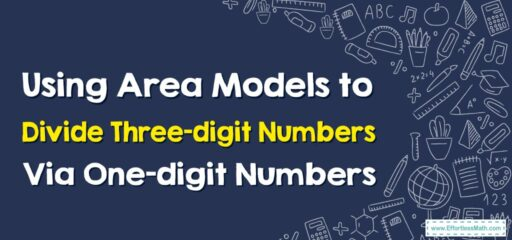# How to Use Area Models to Divide Three-digit Numbers By One-digit Numbers

Using area models to divide three-digit numbers by one-digit numbers can help students visualize and understand the division process.## A Step-by-step Guide to Using Area Models to Divide Three-digit Numbers Via One-digit Numbers

Here’s a step-by-step guide to using area models to divide three-digit numbers by one-digit numbers:

### Step 1: Choose a three-digit dividend and a one-digit divisor.

For example, let’s use 348 divided by 4.

### Step 2: Draw a large rectangle to represent the dividend on a piece of paper.

Divide this rectangle into three vertical sections to represent the hundreds, tens, and ones places.

### Step 3: Label each section with the corresponding place value.

In this case, the hundreds section is 300, the tens section is 40, and the ones section is 8.

### Step 4: Focus on the hundreds section (300).

Divide this section into four equal parts vertically by drawing three vertical lines to split it.

### Step 5: Each part represents 100 ÷ 4 = 25.

Write “25” in each of the four sections within the hundreds area.

### Step 6: Move to the tens section (40).

Divide this section into four equal parts vertically by drawing three vertical lines.

### Step 7: Each part represents 10 ÷ 4 = 2.5.

Since we cannot have half of a section in this model, split two of the sections in half to visually represent 2 each, and leave the remaining two sections as they are.

### Step 8: Move to the ones section (8).

Divide this section into four equal parts vertically by drawing three vertical lines.

### Step 9: Each part represents 1 ÷ 4 = 0.25.

Since we cannot accurately represent 0.25 visually in this model, leave the ones section as a whole without splitting it further.

### Step 10: Count the total number of sections in the hundreds, tens, and ones areas.

In this case, there are 25 sections in the hundreds area, 10 sections in the tens area, and 2 sections in the ones area.

### Step 11: Write down the division steps

$$348÷4=87$$. Each section represents one unit, so the quotient is 87.

Using area models helps students visualize the division process and understand how a three-digit number is divided by a one-digit number. It allows them to see the relationship between place values and the concept of division.

### What people say about "How to Use Area Models to Divide Three-digit Numbers By One-digit Numbers - Effortless Math: We Help Students Learn to LOVE Mathematics"?

No one replied yet.

X
30% OFF

Limited time only!

Save Over 30%

SAVE $5 It was$16.99 now it is \$11.99